# AP Physics C: Mechanics : Understanding Gravity

## Example Questions

### Example Question #1 : Gravity

A block with a mass ofis traveling at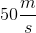when it impacts the ground. From how many meters off the ground was the block dropped?

Round to the nearest whole number.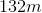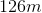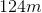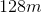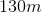Explanation:

Set the gravitational potential energy and kinetic energy equal to each other and solve for the height.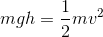Mass cancels.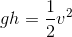Isolate the height and plug in our values.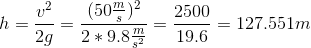Rounding this gives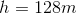.

### Example Question #21 : Forces

An object of massis dropped from a tower. The object's drag force is given by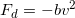whereis a positive constant. What will the objects terminal velocity be?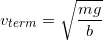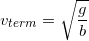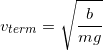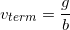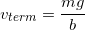Explanation:

To find terminal velocity, set the magnitude of the drag force equal to the magnitude of the force of gravity since when these forces are equal and opposite, the object will stop accelerating: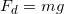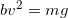Solve for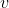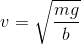### Example Question #22 : Forces

A ship of massand an initial velocity of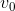is coasting to a stop. The water exerts a drag force on the ship. The drag force is proportional to the velocity: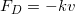where the negative sign indicates that the drag force acts in a direction opposite the motion. After the ship has coasted for a time equal to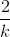, how fast (in terms of) will the ship be moving?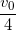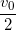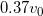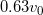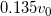Explanation:

The ship's equation of velocity (found by solving the first-order differential equation) is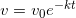Substitute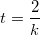and solve.

### Example Question #23 : Forces

A spherical asteroid has a hole drilled through the center as diagrammed below: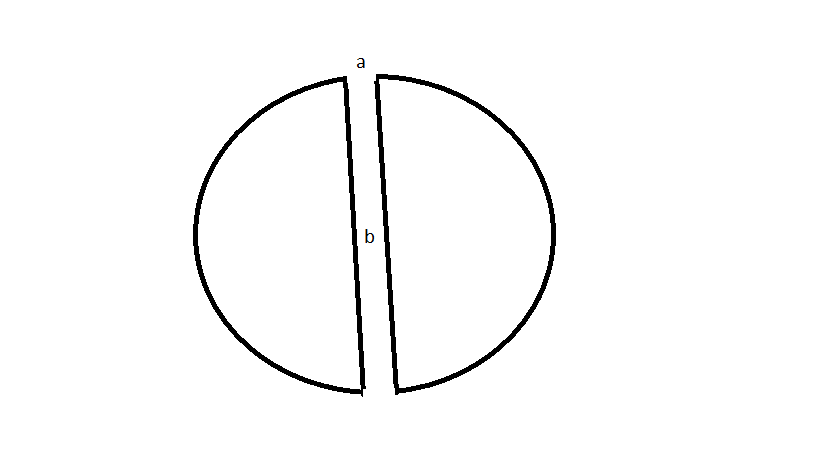Refer to the diagram above. An object that is much smaller than the asteroid is released from rest at the surface of the asteroid, at point a. How do the velocity and acceleration of the object compare at point b at the surface, and point a, located at the center of the asteroid?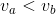and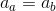and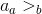and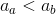and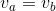andand